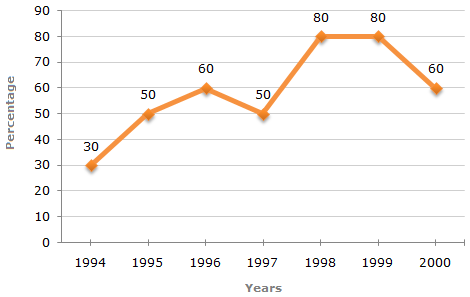# Data Interpretation - Line Charts - Discussion

### Discussion :: Line Charts - Line Chart 5 (Q.No.5)

The following line graph gives the percentage of the number of candidates who qualified an examination out of the total number of candidates who appeared for the examination over a period of seven years from 1994 to 2000.

Percentage of Candidates Qualified to Appeared in an Examination Over the Years5.

The total number of candidates qualified in 1999 and 2000 together was 33500 and the number of candidates appeared in 1999 was 26500. What was the number of candidates in 2000?

 [A]. 24500 [B]. 22000 [C]. 20500 [D]. 19000

Explanation:

The number of candidates qualified in 1999 = (80% of 26500) = 21200.Number of candidates qualified in 2000 = (33500 - 21200) = 12300.

Let the number of candidates appeared in 2000 be x.

 Then, 60% of x = 12300x =12300 x 100= 20500. 60

 Todje said: (Oct 5, 2011) The number of students that qualified is LESS than the number of students who appeared (that is, those who turned up to take the exam they were eligible for). This makes no sense!?

 Hardik Chawda said: (Apr 19, 2015) Please Resolve my Doubts. x = Digital Sum(12300) X D.Sum(100)/D.Sum(60). = D.Sum(6X1)/D.Sum(6) = 1. Eliminate click option D in which case D, sum should be used.

 Jyothi said: (Feb 11, 2017) Can you please explain me with detailed how 80% of 26500=21200? I did not understand this step.

 Balaji Balaji said: (Apr 13, 2017) 80/100 * 26500 = 21200.

 Aditi said: (Aug 8, 2019) 26500*80/100 = 21200.

 Mohammd Aiyoob said: (Jan 12, 2020) Can you please explain to me with detailed how 80% of 26500=21200? I did not understand this step.

 Deepu said: (Mar 16, 2020) First know the formula, Percentage = (Qualified /Appeared)x 100. Given in 1999 candidates appeared was 26500 and from graph percentage in 1999 is 80 Therefore using above formula, 80 = (Q/26500)x 100. Q in 1999 =21200. And also given candidate qualified in both years is 33500. Therefore, Q(1999)+Q(2000) = 33500. Q(2000)=33500-21200 = 12300. They asked to find number of candidates in 2000 means they are asking appeared candidates Again use above percentage formula, 60 = (12300/A)x100. A = 20500.